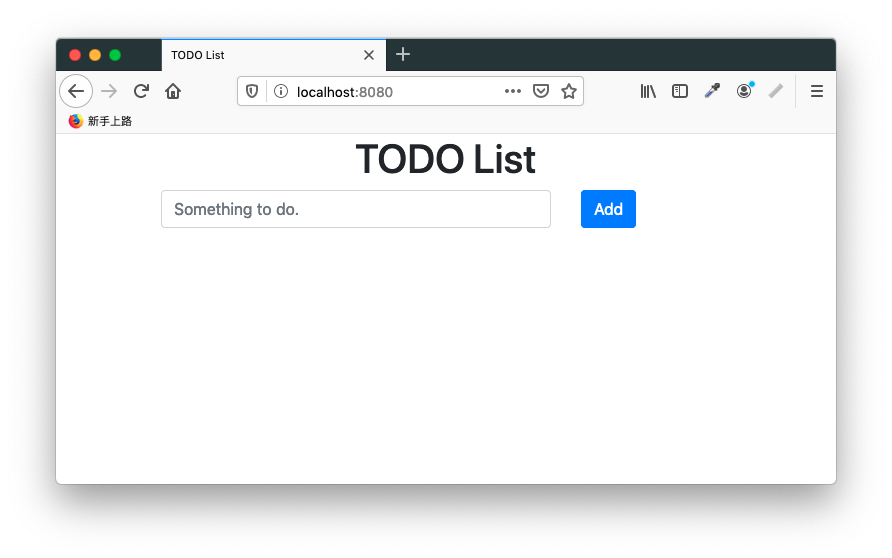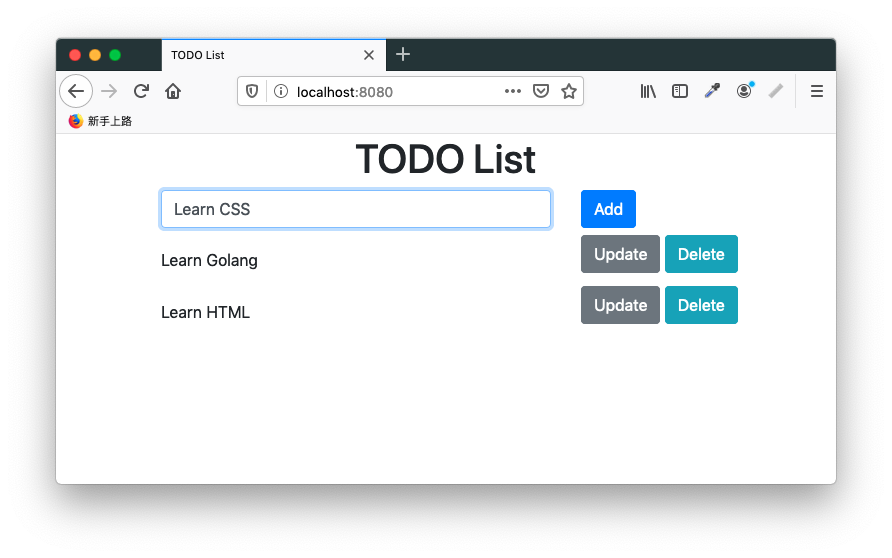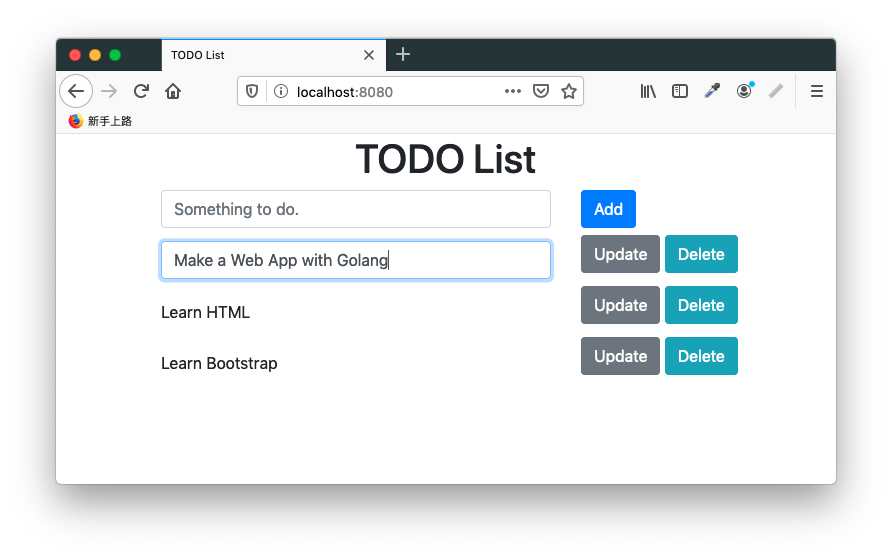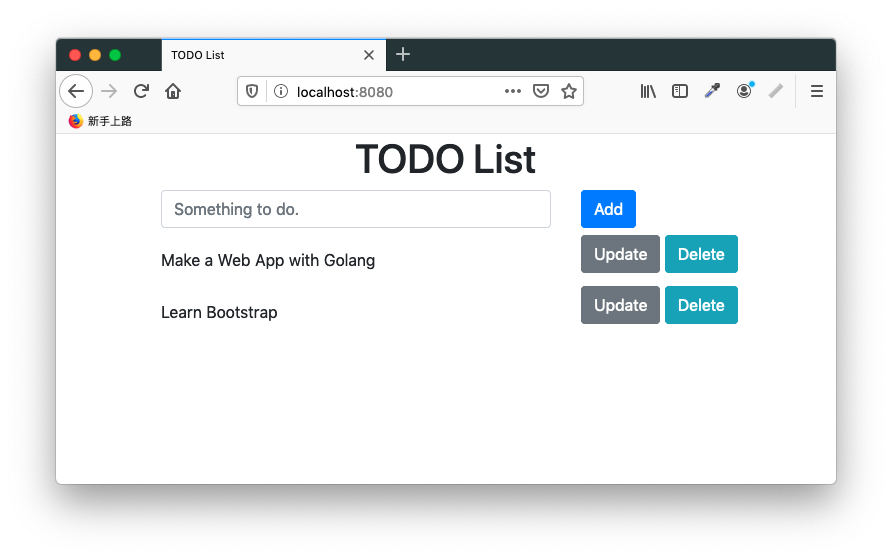# 撰寫 HTML 表單 (Form)

## 前言

在傳統上，網站會使用 HTML 表單 (HTML form) 和網站使用者互動。一些互動的實例像是填寫問卷、填寫量表、填寫報名表等。HTML 表單往往會搭配網頁後端程式來傳輸資料，以及使用資料庫 (database) 儲存資料。

由於 HTML 表單在送出資料時會重刷整個頁面，需要耗費額外的網頁頻寬，對於使用者體驗也不是那麼好。新式的網頁程式較少使用 HTML 表單傳輸資料，而會用 Ajax 等非同步的方式來傳送資料，減少頁面重刷的次數。

在本文中，我們仍然會展示 HTML 表單的撰寫方式。雖然 HTML 表單算是傳統的網頁技術，對於簡易的線上表格來說，使用 HTML 表單仍是最簡單的方式。

## 展示本範例程式

本範例程式是一個 TODO 清單程式。TODO 清單相當適合用來當成練功的題目。對於網頁程式來說，在撰寫 TODO 清單程式時，前端、後端、資料庫都用上了，可說是相當全面的練習。網路上就有強者以各種網頁框架寫 TODO 網頁程式，有興趣的讀者可以前往觀摩一下。

本程式第一次開啟時，TODO 清單是空的：我們可以逐一地加入新的 TODO 項目：我們可以修改 TODO 項目的內容：當項目完成時，我們可以刪除該 TODO 項目：讀者可以自行下載本範例程式，實際把玩一下，比較會有具體的感受。

## 注意事項

為了免除讀者設置資料庫的負擔，本範例程式使用 SQLite，並將該資料庫存在記憶體中。這種形式的資料庫，不會在硬碟中留下實體資料，相當適合用於展示用途。但每次關閉程式時，資料就會歸零，所以實務上不適合使用這種方式儲存資料。

## 專案程式碼

由於這個範例的程式碼較多，我們不會把所有的程式碼都貼上來，這樣子文章篇幅會過長。我們把完整的範例專案放在這裡，有興趣的讀者可以自行追蹤程式碼。我們會在文章中節錄一部分程式碼，便於說明。

## 起始畫面

由於這個程式規模很小，我們只用單一頁面做為程式的界面。我們將頁面放在網頁程式的根目錄，在 Golang 網頁程式中，要將 `indexHandler` 註冊到根目錄：

``````mux.GET("/", indexHandler)
``````

以下是 `indexHandler` 部分的程式碼：

``````func indexHandler(
w http.ResponseWriter,
r *http.Request,
p httprouter.Params) {
var tmpl = template.Must(
template.ParseFiles(
"views/layout.html",
"views/index.html",
"views/head.html"),
)

var msg string

if r.Header.Get("Message") != "" {
msg = r.Header.Get("Message")

// Clean current message.
r.Header.Set("Message", "")
}

rows, err := db.Table("todos").Select("*").Rows()
if err != nil {
msg = "Unable to retrieve database"
}

var todos []TODO

todos = make([]TODO, 0)

for rows.Next() {
var todo struct {
ID   uint
Todo string `gorm:"todo"`
}

db.ScanRows(rows, &todo)

todos = append(todos, TODO{
Index: todo.ID,
Item:  todo.Todo,
})
}

data := struct {
Title   string
TODOs   []TODO
Message string
}{
Title:   "TODO List",
TODOs:   todos,
Message: msg,
}

err = tmpl.ExecuteTemplate(w, "layout", data)
if err != nil {
http.Error(w, err.Error(), 500)
}
}
``````

一開始，我們先載入網頁模板。由於模板是固定的，不應出現載入失敗的情形，故我們直接用 template.Must() 函式載入模板，該函式在載入模板失敗時會直接引發 panic 以中止程式。

當網頁程式出現錯誤時，不能直接把程式中止掉，而要用友善的訊息提示使用者。我們檢查網頁程式的 header 中是否有錯誤訊息，若有錯誤訊息則將其存到程式中，並清空舊訊息。這個錯誤訊息是我們自己塞入 header 中的。

接下來，我們的網頁程式和資料庫互動，取出現有的 TODO 項目：

``````rows, err := db.Table("todos").Select("*").Rows()
if err != nil {
msg = "Unable to retrieve database"
}

var todos []TODO
todos = make([]TODO, 0)

for rows.Next() {
var todo struct {
ID   uint
Todo string `gorm:"todo"`
}

db.ScanRows(rows, &todo)

todos = append(todos, TODO{
Index: todo.ID,
Item:  todo.Todo,
})
}
``````

在這裡，我們沒有用原生的 SQL 語法，而是藉由 GORM 間接呼叫資料庫。

`db.Table("todos").Select("*").Rows()` 會回傳資料庫對表格 `todos` 查詢 (query) 的結果，這時資料庫游標 (cursor) 會停留在查詢結果的第一行 (record)。我們接著以 `rows.Next()` 逐一走訪查詢結果的每一行。以 `db.ScanRows(rows, &todo)` 將該行的資料掃出來。

最後，我們將資料傳入模板，就會是網站使用者實際看到的頁面。雖然一開始 TODO 項目是空的，但我們的範例使用 HTML 表單製作，網站使用者在使用網站的過程中此網頁程式會重刷頁面多次，故我們這些程式碼不會做白工。

有關模板的部分請看這裡，此處不重覆展示。

另外，我們在網頁前端註冊了幾個事件處理器，這是為了讓網頁程式操作起來更便利。我們不會每個小動作都重刷頁面，而會把一些功能做在前端。

我們為每個 TODO 項目註冊了 `click` 事件處理器，在按到某個 TODO 項目時，該 TODO 項目所在的 HTML 標籤會從 `<label>` 轉為 `<input>`，便於網站使用者輸入資料。參考程式碼如下：

``````for (let i = 0; i < todos.length; i++) {
todos[i].addEventListener('click', function () {
for (let j = 0; j < todos.length; j++) {
let label = todos[j].querySelector('label');

if (i === j) {
if (label) {
let text = label.innerText;

let input = document.createElement('input');

input.classList.add('form-control');
input.name = 'todo';
input.value = text;

let index = todos[j].querySelector('[name="index"]').getAttribute('value');

let inputIndex = document.createElement('input');

inputIndex.setAttribute('value', index);
inputIndex.name = 'index'
inputIndex.setAttribute('hidden', true);

todos[i].innerHTML = '';
todos[i].appendChild(input);
todos[i].appendChild(inputIndex);
}
} else {
if (!label) {
let input = todos[j].querySelector('input');

let text = input.value;

let label = document.createElement('label');

label.classList.add('col-form-label');
label.innerText = text;

let index = todos[j].querySelector('[name="index"]').getAttribute('value');

let inputIndex = document.createElement('input');

inputIndex.setAttribute('value', index);
inputIndex.name = 'index'
inputIndex.setAttribute('hidden', true);

todos[j].innerHTML = '';
todos[j].appendChild(label);
todos[j].appendChild(inputIndex);
}
}
}
});
}
``````

在這段程式碼中，我們使用兩個 `for` 迴圈。外部的 `for` 迴圈位於事件處理器外，是為了走訪每個 TODO 項目。內部的 `for` 迴圈位於事件處理器中，是為了在按下 TODO 項目時，將按下的 TODO 項目由 `<label>` 轉為 `<input>`，並將其他的 TODO 項目由 `<input>` 轉回 `<label>`

我們在這裡沒有使用額外的前端框架，完全用原生的網頁 API 處理動態生成界面的部分。使用原生網頁 API 的好處在於不用受限於前框框架的 API，可以自由地按照自己的想法去寫網頁程式。但用原生網頁 API 寫起來會比較長一些。

我們另外幫頁面註冊一個 ESC 鈕的事件處理器，在按下 ESC 鈕時會將頁面上所有的 TODO 項目的 `<input>` 元素轉回 `<label>` 元素。參考以下程式碼：

``````document.addEventListener('keydown', function (event) {
if (event.which === 27) {
for (let i = 0; i < todos.length; i++) {
let label = todos[i].querySelector('label');

if (!label) {
let input = todos[i].querySelector('input');

let text = input.value;

let label = document.createElement('label');

label.classList.add('col-form-label');
label.innerText = text;

let index = todos[i].querySelector('[name="index"]').getAttribute('value');

let inputIndex = document.createElement('input');

inputIndex.setAttribute('value', index);
inputIndex.name = 'index'
inputIndex.setAttribute('hidden', true);

todos[i].innerHTML = '';
todos[i].appendChild(label);
todos[i].appendChild(inputIndex);
}
}
}
})
``````

這些功能和使用者界面相關，所以會用 JavaScript 寫在網頁前端，而不會用 Golang 寫在網頁後端。

## 新增 TODO 項目

在 HTML 表單中，會使用 `<form>` 網頁元素將表單的部分包起來。以本範例程式的第一個表單來看：

``````<form action='/todo/' method='POST'>
<div>
<div>
<input type='text' name='todo' placeholder='Something to do.'>
</div>

<div>
<button>Add</button>
</div>
</div>
</form>
``````

在撰寫表單時，我們會指定表單的行為 (action) 和動作 (method)。行為對應到網頁程式的路徑 (route)；動作則是 HTTP 請求方法，在此範例中我們使用 POST 。當網站使用者傳送表單時，瀏覽器會依據我們指定的行為和動作呼叫網頁程式中相對應的路徑和請求方法。

在網頁程式中也要註冊相對應的事件處理器：

``````mux.POST("/todo/", updateTODOHandler)
``````

以下是事件處理器的 Golang 程式碼：

``````func updateTODOHandler(
w http.ResponseWriter,
r *http.Request,
p httprouter.Params) {
r.ParseForm()

todo := r.FormValue("todo")
method := r.FormValue("_method")

if todo == "" {
r.Header.Set("Message", "Empty TODO item")
} else if method == "update" {
// Implement it later.
} else if method == "delete" {
// Implement it later.
} else {
db.Table("todos").Create(struct {
Todo string `gorm:"todo"`
}{
Todo: todo,
})
}

http.Redirect(w, r, "/", http.StatusSeeOther)
}
``````

細心的讀者應可發現我們將一部分程式碼註解掉，這和瀏覽器的限制有關，我們會在後文詳談。

一開始我們以 `r.ParseForm()` 解析表單，再以 `todo := r.FormValue("todo")` 取出使用者寫入的 TODO 項目。這個值會由頁面上的 `<input type='text' name='todo' placeholder='Something to do.'>` 網頁元素的值來決定，串連的方式是由網頁元素的 `name` 屬性來決定。

`todo` 為空時，我們會在網頁 header 中寫入錯誤訊息。若 `todo` 確實有值，我們將其寫入資料庫：

``````db.Table("todos").Create(struct {
Todo string `gorm:"todo"`
}{
Todo: todo,
})
``````

這裡同樣用 GORM 間接呼叫資料庫。

最後，我們把頁面重導 (redirect) 到首頁：

``````http.Redirect(w, r, "/", http.StatusSeeOther)
``````

為什麼我們在這裡要重導網址呢？對於網站客戶端來說，傳送表單等同於發出新的請求 (request)，發出請求後，網頁程式得回傳新的頁面，否則使用者看到的就是空白的頁面。但我們想重覆利用同一個首頁來當使用者介面，故我們利用重導將網頁客戶端引導回網站的首頁。

## 更新 TODO 項目

在我們的範例程式中，每個 TODO 項目其實都是一個獨立的 HTML 表單。由於 TODO 項目有多個，我們使用 Golang 模板語言的 `range` 當成迴圈來生成多個 HTML 表單。模板部分的程式碼如下：

``````{{ range \$t := .TODOs }}
<form action='/todo/' method='POST'>
<div>
<div class='todo'>
<label>{{ \$t.Item }}</label>
<inupt name='index' name='index' value='{{ \$t.Index }}' hidden>
</div>

<div>
<button type='submit' name='_method' value='update' onclick="updateTODO(event);">Update</button>
<button type='submit' name='_method' value='delete' onclick="deleteTODO(event);">Delete</button>
</div>
</div>
</form>
{{ end }}
``````

細心的讀者應該已經發現我們的表單的行為和方法同樣是 `/todo/`POST 。我們在先前已經使用過這組行為和方法了，為什麼還要重覆使用呢？這牽涉到瀏覽器的限制。簡單地說，透過瀏覽器傳送的表格，只能用 GETPOSTdialog 等 HTTP 請求方法。

這時候，我們會用一些手法來處理這項議題。關鍵的按鈕元素如下：

``````<button type='submit' name='_method' value='update' onclick="updateTODO(event);">Update</button>
<button type='submit' name='_method' value='delete' onclick="deleteTODO(event);">Delete</button>
``````

我們在同一個表格中有兩個按鈕，勢必要對應到不同的行為。我們刻意地將兩個按鈕指定到相同的 `name` 屬性，並分別在 `onclick` 事件對應到不同的函式。本節會看更新 TODO 項目的方法。

我們在 `onclick` 事件指向以下函式：

``````function updateTODO (event) {
let form = event.target.parentNode.parentNode.parentNode;

let todo = form.querySelector('.todo');

let label = todo.querySelector('label');

if (label) {
let text = label.innerText;

let input = document.createElement('input');

input.classList.add('form-control');
input.name = 'todo';
input.value = text;

let index = todo.querySelector('[name="index"]').getAttribute('value');

console.log(todo.querySelector('[name="index"]'));
console.log(`index: \${index}`);

let inputIndex = document.createElement('input');

inputIndex.setAttribute('value', index);
inputIndex.name = 'index'
inputIndex.setAttribute('hidden', true);

todo.innerHTML = '';
todo.appendChild(input);
todo.appendChild(inputIndex);
}

form.setAttribute('_method', 'update');
form.submit();
}
``````

這個函式在按下時，會先將 TODO 項目的 `<label>` 元素轉為 `<input>` 元素，因為 `<input>` 元素才能傳送到網頁後端程式。

接著，關鍵的地方在於我們指定 `form` 元素的 `_method` 屬性：

``````form.setAttribute('_method', 'update');
``````

當我們傳送表單時，該屬性的值會設為 `update`

我們來看網頁後端程式相對應的事件處理器：

``````func updateTODOHandler(
w http.ResponseWriter,
r *http.Request,
p httprouter.Params) {
r.ParseForm()

todo := r.FormValue("todo")
method := r.FormValue("_method")

if todo == "" {
r.Header.Set("Message", "Empty TODO item")
} else if method == "update" {
index := r.FormValue("index")

if index == "" {
r.Header.Set("Message", "Unable to retrieve TODO item")
} else {
db.Table("todos").Where("id == ?", index).Update(struct {
Todo string `gorm:"todo"`
}{
Todo: todo,
})
}
} else if method == "delete" {
// Implement it later.
} else {
// Create a TODO item.
}

http.Redirect(w, r, "/", http.StatusSeeOther)
}
``````

我們以 `r.FormValue("_method")` 讀入我們先前指定的 `_method` 屬性，當該屬性的值為 `"update"` 時，更新資料庫中相對應的 TODO 項目。

我們怎麼知道頁面上的 TODO 項目在資料庫中所在的位置？因為我們在頁面中以一個額外的隱藏 `<input>` 元素儲存該 TODO 項目在資料庫中相對應的位置。

## 刪除 TODO 項目

我們在更新和刪除 TODO 項目時，重覆使用同一個 HTML 表單，所以表單的模板基本上是重覆的：

``````{{ range \$t := .TODOs }}
<form action='/todo/' method='POST'>
<div>
<div class='todo'>
<label>{{ \$t.Item }}</label>
<inupt name='index' name='index' value='{{ \$t.Index }}' hidden>
</div>

<div>
<button type='submit' name='_method' value='update' onclick="updateTODO(event);">Update</button>
<button type='submit' name='_method' value='delete' onclick="deleteTODO(event);">Delete</button>
</div>
</div>
</form>
{{ end }}
``````

關鍵的地方在於我們在 `Delete` 按鈕指定不同的函式：

``````<button type='submit' name='_method' value='delete' onclick="deleteTODO(event);">Delete</button>
``````

該函式的程式碼如下：

``````function deleteTODO (event) {
let form = event.target.parentNode.parentNode.parentNode;

let todo = form.querySelector('.todo');

let label = todo.querySelector('label');

if (label) {
let text = label.innerText;

let input = document.createElement('input');

input.classList.add('form-control');
input.name = 'todo';
input.value = text;

let index = todo.querySelector('[name="index"]').getAttribute('value');

console.log(todo.querySelector('[name="index"]'));
console.log(`index: \${index}`);

let inputIndex = document.createElement('input');

inputIndex.setAttribute('value', index);
inputIndex.name = 'index'
inputIndex.setAttribute('hidden', true);

todo.innerHTML = '';
todo.appendChild(input);
todo.appendChild(inputIndex);
}

form.setAttribute('_method', 'delete');
form.submit();
}
``````

其實大部分的程式碼是重覆的，主要的差別在於我們把 `_method` 屬性設為 `delete`

在網頁後端程式的部分，我們也是重覆使用相同的事件處理器，但實際執行的區塊不同。參考以下程式碼：

``````func updateTODOHandler(w http.ResponseWriter, r *http.Request, p httprouter.Params) {
r.ParseForm()

todo := r.FormValue("todo")
method := r.FormValue("_method")

if todo == "" {
r.Header.Set("Message", "Empty TODO item")
} else if method == "update" {
// Update a TODO item.
} else if method == "delete" {
index := r.FormValue("index")

if index == "" {
r.Header.Set("Message", "Unable to retrieve TODO item")
} else {
db.Table("todos").Where("id == ?", index).Delete(struct {
ID   uint
Todo string
}{})
}
} else {
// Create a TODO item.
}

http.Redirect(w, r, "/", http.StatusSeeOther)
}
``````

我們用 `r.FormValue("_method")` 讀入表單的 `_method` 屬性，當該屬性為 `delete` 時，觸發相關的資料庫動作，將該 TODO 項目刪除。

藉由這項手法，我們在原有的 POST 方法外，另外模擬出 PUTDELETE 兩種 HTTP 請求方法。這並不是好的模式 (pattern)，而是因瀏覽器的限制不得不的權宜之計。

## 結語

在本範例程式中，我們以經典的 TODO 清單程式展示 HTML 表單的撰寫方法。在練習的過程中，我們練習到網頁前端、網頁後端、資料庫等多個面向，對於網頁程式來說，這是一個很好的練習題材。

使用 HTML 表單，算是比較傳統的手法。但 HTML 表單需要多次重刷頁面，對使用者體驗不是很好。在後續的文章中，我們會用 Ajax 來改善這個程式。

【分享本文】